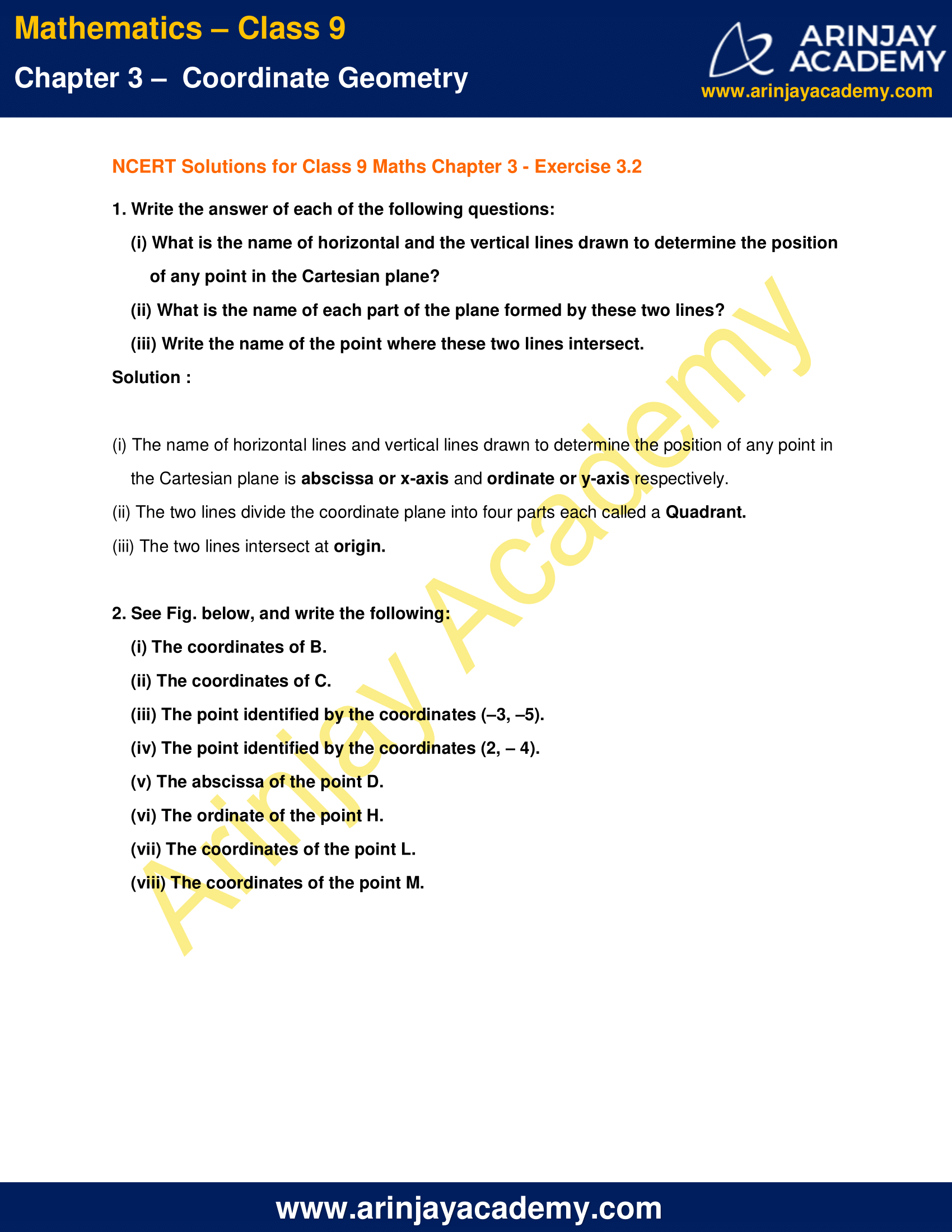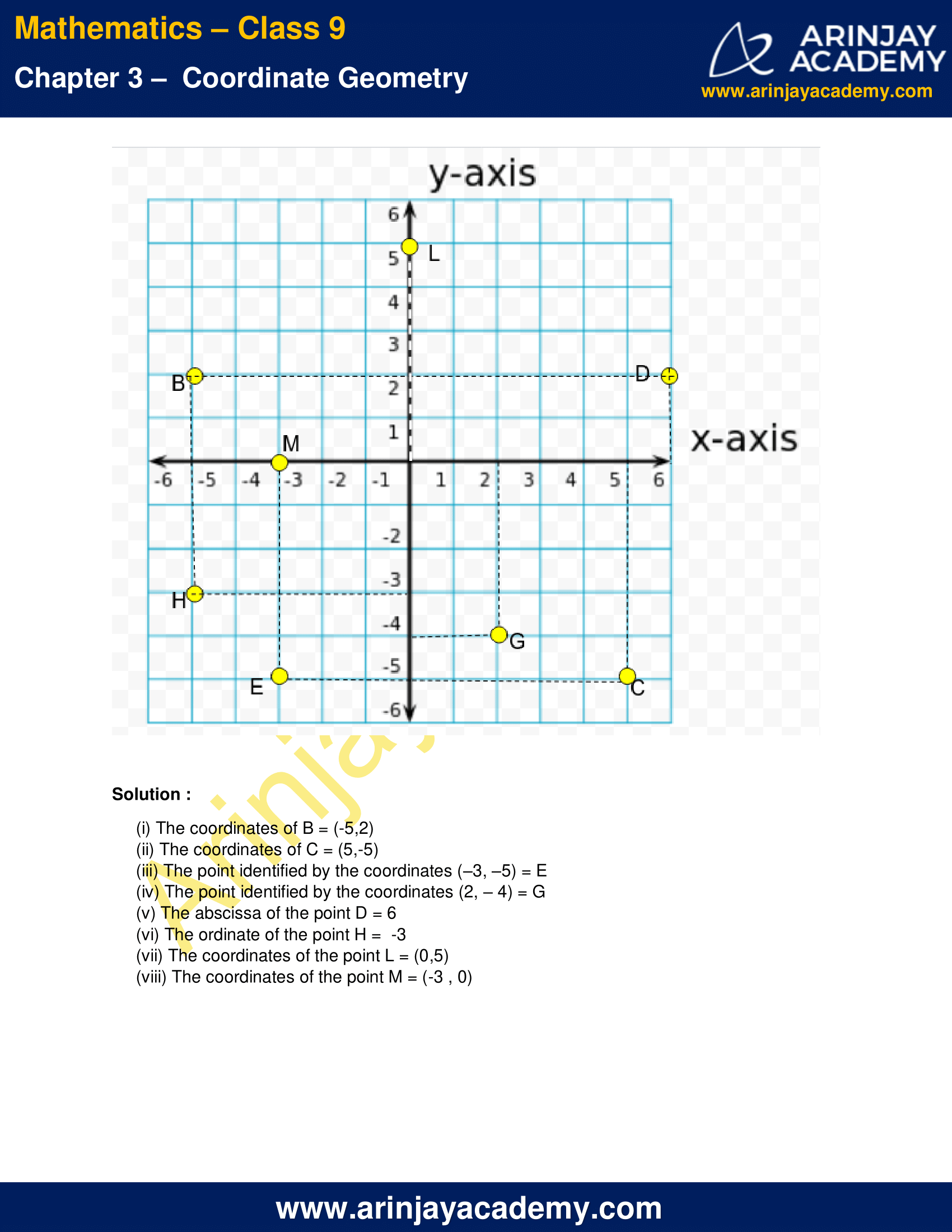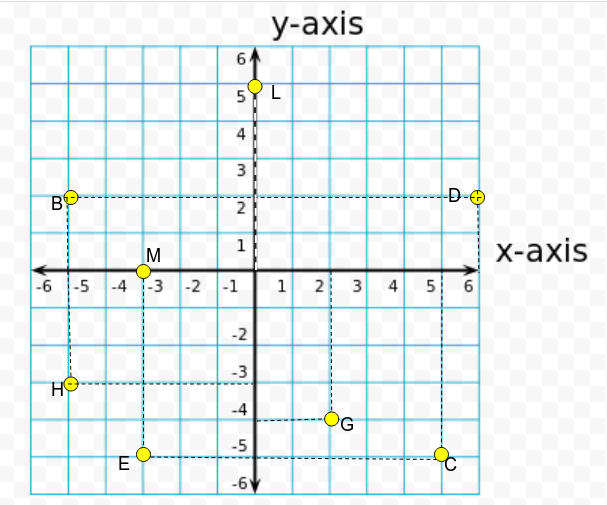# NCERT Solutions for Class 9 Maths Chapter 3 Exercise 3.2 Coordinate Geometry

Download NCERT Solutions for Class 9 Maths Chapter 3 Exercise 3.2 Coordinate Geometry. This Exercise contains 2 questions, for which detailed answers have been provided in this note. In case you are looking at studying the remaining Exercise for Class 9 for Maths NCERT solutions for Chapter 3 or other Chapters, you can click the link at the end of this Note.

### NCERT Solutions for Class 9 Maths Chapter 3 Exercise 3.2 Coordinate GeometryNCERT Solutions for Class 9 Maths Chapter 3 Exercise 3.2 Coordinate Geometry

1. Write the answer of each of the following questions:

(i) What is the name of horizontal and the vertical lines drawn to determine the position of any point in the Cartesian plane?
(ii) What is the name of each part of the plane formed by these two lines?
(iii) Write the name of the point where these two lines intersect.

Solution :

(i) The name of horizontal lines and vertical lines drawn to determine the position of any point in the Cartesian plane is abscissa or x-axis and ordinate or y-axis respectively.
(ii) The two lines divide the coordinate plane into four parts each called a Quadrant.
(iii) The two lines intersect at origin.

2. See Fig. below, and write the following:
(i) The coordinates of B.
(ii) The coordinates of C.
(iii) The point identified by the coordinates (–3, –5).
(iv) The point identified by the coordinates (2, – 4).
(v) The abscissa of the point D.
(vi) The ordinate of the point H.
(vii) The coordinates of the point L.
(viii) The coordinates of the point M.Solution :

(i) The coordinates of B = (-5,2)
(ii) The coordinates of C = (5,-5)
(iii) The point identified by the coordinates (–3, –5) = E
(iv) The point identified by the coordinates (2, – 4) = G
(v) The abscissa of the point D = 6
(vi) The ordinate of the point H =  -3
(vii) The coordinates of the point L = (0,5)
(viii) The coordinates of the point M = (-3 , 0)

NCERT Solutions for Class 9 Maths Chapter 3 Exercise 3.2 – Coordinate Geometry, has been designed by the NCERT to test the knowledge of the student on the topic – Cartesian System

The next Exercise for NCERT Solutions for Class 9 Maths Chapter 3 Exercise 3.3 – Coordinate Geometry can be accessed by clicking here.

Download NCERT Solutions for Class 9 Maths Chapter 3 Exercise 3.2 Coordinate Geometry